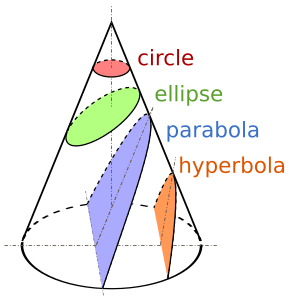Conic section facts for kids

Kids Encyclopedia FactsConics are of three types: parabolas, ellipses, including circles, and hyperbolas

In geometry, when a cone and a plane intersect, a conic section results.

Preliminary
In elementary geometry, cones are assumed to be right circular. Circular means that the base is a circle and right means that the axis passes through the centre of the base at right angles to its plane. Contrasted with right cones are oblique cones, in which the axis does not pass perpendicularly through the centre of the base.
Definition
A conic is the curve got by intersecting a plane, called the cutting plane, with a cone. The cone is a right circular cone for easy description, but any double cone with some circular cross-section will do.

There are three different types of sections, the parabola, the hyperbola and the ellipse. The circle is a special case of an ellipse. Sometimes the circle is mentioned as a fourth type, because calculating with a circle is easier than with an ellipse, most of the time.

The conic sections are known at least since 200 BC, when Apollonius of Perga (~262 BC to ~190 BC) studied of their properties. They came to more attention when mathematicians discovered that the solutions of the two-body problem were conic sections.

Images for kidsConic section Facts for Kids. Kiddle Encyclopedia.